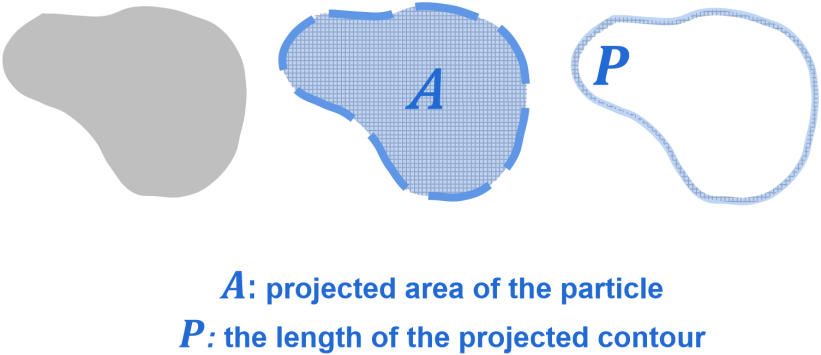Hmoe > Learn > Wiki > How to calculate the projected area and the contour of a particle?

# How to calculate the projected area and the contour of a particle?

2023-07-17WIKI

Area, in this instance, refers to the projected area of a particle. The projected area of a particle can be calculated by multiplying the number of pixels by the area of a pixel. The area of a pixel can be calculated with the help of the calibration coefficient.

The perimeter of a particle projection refers to the total length of its projected contour. The perimeter can be calculated through contour representation using curve fitting with the help of an algorithm.Struggling to grasp particle analysis concepts? Our new Beginner's Guide to Particle Analysis is here to help you grasp these concepts in the most accessible manner possible. Download it as a PDF to read on the train, on the plane, or wherever else there’s no internet.